# Getting to the Bottom of Regression with Gradient Descent

## Features

• Author: Jocelyn T. Chi and Eric C. Chi
• Date: 10 Jan 2014
• Copyright: Image appears courtesy of iStock Photo

This article on gradient descent was written as part of a series on optimization methods for Statisticsviews.com. The article presents an overview of the gradient descent algorithm, offers some intuition on why the algorithm works and where it comes from, and provides examples of implementing it for ordinary least squares and logistic regression in R.

## Introduction

From splines to generalized linear models, many problems in statistical estimation and regression can be cast as optimization problems. To identify trends and associations in data, we often seek the “best” fitting curve from a family of curves to filter the noise from our signals. In this article, we will consider “best” to be in terms of maximizing a likelihood.

Consider, for example, ordinary least squares (OLS) regression. OLS regression amounts to finding the line, or more generally, the plane, with minimal total Euclidean distance to the data points. In other words, we seek the plane that minimizes the distance between the data points and their orthogonal projections onto the plane. Formally, we seek a regression vector b that minimizes the objective or loss function $\begin{equation} \label{eq:min_problem} f({\bf b}) = \frac{1}{2} || {\bf y} - {\bf X}{\bf b} ||^{2}, \end{equation}$ where y is a vector of response variables and X is the design matrix. Although we can also obtain the solution to OLS regression directly by solving the normal equations, many other estimation and regression problems cannot be answered by simply solving a linear system of equations. Moreover, even solving a linear system of equations can become non-trivial when there are many parameters to be fit. Thus, we are motivated towards a simple approach to solving optimization problems that is general and works for a wide range of objective functions.

In this article, we review gradient descent, one of the simplest numerical optimization algorithms for minimizing differentiable functions. While more sophisticated algorithms may be faster, gradient descent is a reliable option when there is a need to fit data with a novel model, or when there are many parameters to be fit. Additionally, gradient descent presents a basis for many powerful extensions, including stochastic and proximal gradient descent. The former enables fitting regression models in very large data mining problems, and the latter has been successfully applied in matrix completion problems in collaborative filtering and signal processing. Understanding how gradient descent works lays the foundation for implementing and characterizing these more sophisticated variants.

In the rest of this article, we will outline the basic gradient descent algorithm and give an example of how it works. Then we will provide some intuition on why this algorithm works and discuss implementation considerations. We end with three examples: two OLS problems, and a logistic regression problem.#### The Basic Algorithm.

Let $$f({\bf b})$$ denote the function we wish to minimize. Starting from some initial point $${\bf b}_0$$, the gradient descent algorithm repeatedly performs two steps to generate a sequence of parameter estimates $${\bf b}_1, {\bf b}_2, \dots$$. At the $$m^{\text{th}}$$ iteration, we calculate $$\nabla f({\bf b_{m}})$$, the gradient of $$f({\bf b})$$ at $${\bf b}_m$$, and then take a step in the opposite direction. Combining these two steps, we arrive at the following update rule to go from the $$m^{\text{th}}$$ iterate to the $$m+1^{\text{th}}$$ iterate: $\begin{equation*} {\bf b}_{m+1} \gets {\bf b}_m - \alpha \nabla f({\bf b}_m), \end{equation*}$ where $$\alpha$$ is a step-size that controls how far we step in the direction of the $$-\nabla f({\bf b_{m}})$$. In principle, we repeatedly invoke the update rule until the iterate sequence converges, since a fixed point $${\bf b}^\star$$ of the update rule is a stationary point of $$f({\bf b})$$, namely $$\nabla f({\bf b}^\star) = 0$$. In practice, we stop short of convergence and run the algorithm until the Euclidean norm of $$\nabla f({\bf b}_m)$$ is sufficiently close to zero. In our examples, we stop the algorithm once $$\lVert \nabla f({\bf b}_m) \rVert \leq 1 \times 10^{-6}$$.

We notice that the gradient descent algorithm stops when the iterates are sufficiently close to a stationary point rather than the global minimizer. In general, stationarity is a necessary but not sufficient condition for a point to be a local minimizer of a function. Convex functions constitute an important exception since all local minima are global minima for convex functions. Thus, finding a global minimizer of a convex function is equivalent to finding a stationary point. For general functions, however, there are no guarantees that gradient descent will arrive at the globally best solution, but this is true for all iterative algorithms.

Later in this article, we will discuss implementation considerations for the gradient descent algorithm, including choosing a step-size $$\alpha$$ and initializing $${\bf b}$$. First, however, let us work through the mechanics of applying gradient descent on a simple example.

#### A Simple Example: Univariate OLS

We made an R package titled “Getting to the Bottom - A Package for Learning Optimization Methods” to enable reproduction of the examples in this article. Use of this package requires R (>= 3.0.2). Once you have R working, you can install and load the package by typing install.packages(gettingtothebottom) into the R console. After loading the package and calling the help file for the gdescent function, the code for each example can be copied and pasted from the help panel into the console.

We start with a simple example of univariate OLS on simulated data. We generate a univariate $${\bf X}$$ of $$50$$ observations with values ranging between $$-1$$ and $$1$$. We then generate a univariate $${\bf y}$$ of $$50$$ observations under the model $$y_{i} = 2x_{i} + \epsilon_{i}$$, where $$\epsilon_{i}$$ iid $$\sim N(0,1)$$.

 # EXAMPLE 1 - A Simple Example library(gettingtothebottom) 

 ## Loading required package: ggplot2  ## Loading required package: grid  

 help(gdescent)   set.seed(12345)  x <- sample(seq(from = -1, to = 1, by = 0.1), size = 50, replace = TRUE)  y <- 2 * x + rnorm(50) 


The figure below shows the data generated for our example.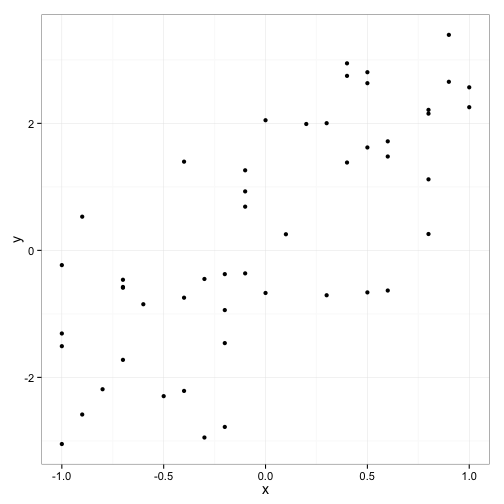To use gradient descent, we need two functions; one to compute the gradient, and one to compute the loss. We use the former to compute the update and the latter to track the progress of the algorithm.

The gradient of $$f$$ at $${\bf b}$$ is \begin{align*} \nabla f({\bf b}) &= {\bf X}^{t}({\bf X}{\bf b} - {\bf y}). \end{align*}

We run our simple example using an initial value of $${\bf b}_0 = {\bf 0}$$, and $$\alpha = 0.01$$. The default setting on the gdescent function automatically adds a column vector of $$1$$'s before the first column in $${\bf X}$$ to estimate an intercept value. If you prefer to withhold the intercept in your model, you can do so by including intercept = FALSE when you call the gdescent function.

The gdescent output returns the minimum value for $$f$$, an intercept, and values for the coefficients from $${\bf b}$$.

 X <- as.matrix(x)  y <- as.vector(y)  f <- function(X, y, b) {      (1/2) * norm(y - X %*% b, "F")^2      }  grad_f <- function(X, y, b) {      t(X) %*% (X %*% b - y)     }  simple_ex <- gdescent(f, grad_f, X, y, 0.01)  

 ## Minimum function value:  ##  36.85  ##  ## Intercept:  ##  0.28  ##  ## Coefficient(s): ##  2.123  


The progress of the algorithm can be observed by calling the plot_loss function.

 plot_loss(simple_ex)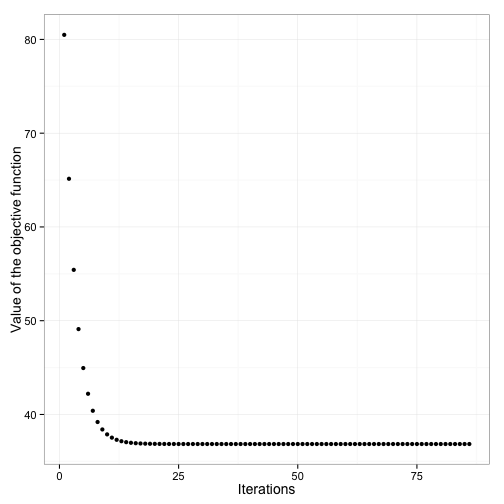The plot shows how the loss function $$f$$ decreases as the algorithm proceeds. The algorithm makes very good progress towards finding a local minimum early on. As it edges closer towards the minimum, however, progress becomes significantly slower.

As a sanity check, we can compare our gdescent results with what we obtain from the lm function for the same problem.

lm(y ~ X) 
 ##   ## Call:  ## lm(formula = y ~ X)  ##   ## Coefficients:  ## (Intercept)            X    ##        0.28         2.12  


We observe that gradient descent and the lm function provide the same solution to the least squares problem.

## Some Intuition on Gradient Descent.

Gradient descent attempts to minimize $$f({\bf b})$$ by solving a sequence of easier minimization problems, namely a sequence of simple quadratic approximations to $$f({\bf b})$$. Fix $$\alpha > 0$$ and let $$\tilde{{\bf b}}$$ denote our current iterate. We know from Taylor's theorem that for b close to $$\tilde{{\bf b}}$$, \begin{align*} f({\bf b}) &\approx f(\tilde{{\bf b}}) + \langle \nabla f(\tilde{{\bf b}}), {\bf b} - \tilde{{\bf b}} \rangle + \frac{1}{2\alpha}||{\bf b} - \tilde{{\bf b}}||^{2}. \end{align*} Consider minimizing the quadratic approximation on the right. When we take the derivative of the approximation with respect to $${\bf b}$$ and set it equal to zero, we obtain \begin{align*} \nabla f(\tilde{{\bf b}}) + \frac{1}{\alpha}({\bf b} - \tilde{{\bf b}}) = 0, \end{align*} and solving for $${\bf b}$$ gives us \begin{align*} {\bf b} &= \tilde{{\bf b}} - \alpha \nabla f(\tilde{{\bf b}}), \end{align*} which we recognize is a gradient step with step-size $$\alpha$$. So we see that the gradient update minimizes a quadratic approximation to $$f({\bf b})$$.

When $$\alpha$$ is very large, $$\frac{1}{2\alpha}\lVert {\bf b} - \tilde{{\bf b}}\rVert ^{2}$$ becomes very small and the approximation of $$f$$ at $${\bf b}$$ becomes flat. Conversely, when $$\alpha$$ is very small, $$\frac{1}{2\alpha}\lVert {\bf b} - \tilde{{\bf b}}\rVert ^{2}$$ becomes quite large and correspondingly, the curvature of the approximation becomes more pronounced. The figure below illustrates how the choice of $$\alpha$$ affects the quadratic approximation employed in the algorithm.

example.quadratic.approx(alpha1 = 0.01, alpha2 = 0.12)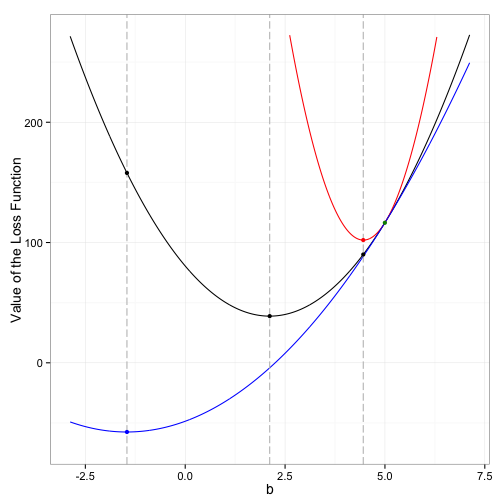In this figure, the black line depicts the OLS objective function $$f$$ that we minimized in Example 1. The green dot denotes an initial starting point $${\bf b}$$ for the gradient descent algorithm. The red and blue curves show the quadratic approximations for $$f$$ when $$\alpha=0.01$$ and $$\alpha=0.12$$, respectively. The dotted vertical lines intersect the curves at their minima. The lines also show how the minima provide different anchor points for the quadratic approximation of $$f$$ in the next iteration of the algorithm.

Intuitively, choosing large values for the step-size $$\alpha$$ results in greater progress towards a minimum in each iteration. As $$\alpha$$ increases, however, the approximation becomes more linear and the minimizer of the approximation can drastically overshoot the minimizer of $$f$$. We can ensure monotonically decreasing objective function values (i.e. $$f({\bf b}_m) \geq f({\bf b}_{m+1}) \geq f({\bf b}_{m+2}) \geq \ldots$$) if the approximations always “sit on top” of $$f$$ (like the red approximation above). This prevents us from wildly overshooting the minimizer. Formally, we can guarantee monotonically decreasing objective function values when $$\nabla f({\bf b})$$ is $$L$$-Lipschitz continuous and $$\alpha \leq 1/L$$. Recall that $$\nabla f({\bf b})$$ is $$L$$-Lipschitz continuous if \begin{align*} \lVert \nabla f({\bf b}) - \nabla f(\tilde{{\bf b}}) \rVert \leq L \lVert {\bf b} - \tilde{{\bf b}} \rVert, \end{align*} for all $${\bf b}$$ and $$\tilde{{\bf b}}$$. When $$f$$ is twice differentiable, this means that the largest eigenvalue of $$\nabla^2 f({\bf b})$$, the Hessian of $$f$$, is no greater than $$L$$ for all $${\bf b}$$. Lipschitz continuous functions have a bound on how rapidly they can vary. So when the gradient of a function is Lipschitz continuous, we know that roughly speaking, the function has a maximum bound on its curvature. Thus, it is possible to find quadratic approximations that always sit on top of the function, as long as we employ a step-size equal to, or less than, the reciprocal of that bound.

In OLS, $$f({\bf b})$$ is twice differentiable and its Hessian is $${\bf X}^t{\bf X}$$, which does not depend on $${\bf b}$$. Therefore, the smallest Lipschitz constant of $$\nabla f$$ is the largest eigenvalue of $${\bf X}^t{\bf X}$$. Naturally, we want to take the biggest steps possible, so if we can compute the Lipschitz constant $$L$$ we set $$\alpha = 1/L$$.

Using the simple example we employed previously, we observe that when our choice of $$\alpha$$ is not small enough, the norm of the gradient will diverge towards infinity and the algorithm will not converge. (Note that the code for this example is provided below but in the interest of space, we do not include the output in this article.)

simple_ex2 <- gdescent(f,grad_f,X,y,alpha=0.05,liveupdates=TRUE) 

The live updates in this example show the norm of the gradient in each iteration and we can see that the norm of the gradient diverges when $$\alpha$$ is not sufficiently small. The following two figures illustrate why this might occur. In the first figure, $$\alpha$$ is sufficiently small so each iteration in the algorithm results in a step towards the minimum, resulting in convergence of the algorithm.

example.alpha(0.01) 
 ## Minimum function value:  ##  38.81  ##   ## Coefficient(s):  ##  2.114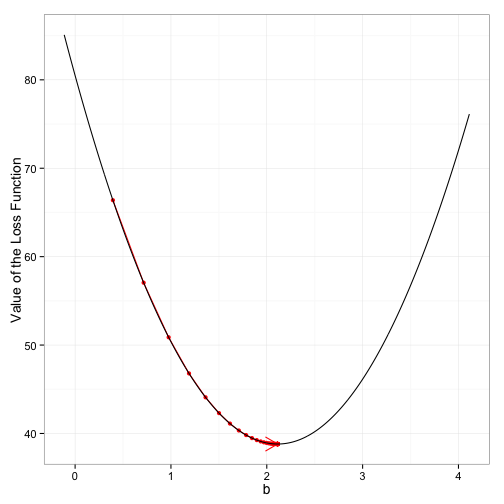In the second figure, $$\alpha$$ is too large and each subsequent iterate increasingly overshoots the minimum, resulting in divergence of the algorithm.

example.alpha(0.12) 
## Minimum function value not attained. A better result might be obtained by decreasing the step size or increasing the number of iterations.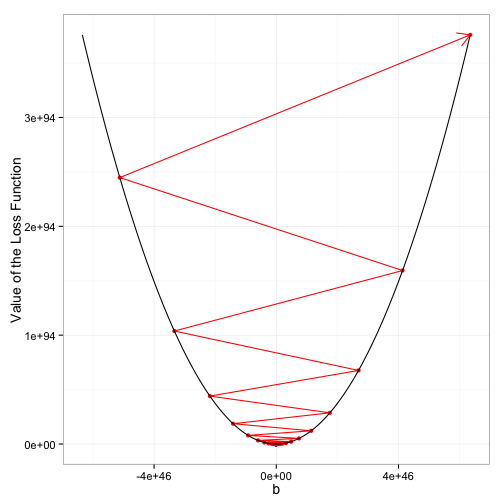In the following section, we discuss some of the decisions required in implementing the gradient descent algorithm.

## Some Implementation Considerations.

#### Choosing a Step-Size Manually

As the figures above indicate, the choice of the step-size $$\alpha$$ is very important in gradient descent. When $$\alpha$$ is too large, the objective function values diverge and the algorithm will not converge towards a local minimum. On the other hand, when $$\alpha$$ is too small, each iteration takes only a tiny step towards the minimum and the algorithm may take a very long time to converge.

A safe choice for $$\alpha$$ is the one derived above using the Lipschitz constant. Sometimes it easy to determine a Lipschitz constant, and the step-size can be appropriately set. But how does one pick an appropriate $$\alpha$$ when the Lipschitz constant is not readily available? In these cases, it is useful to try experimenting with step-sizes. If you know that the objective function has a Lipschitz continuous gradient but do not know the Lipschitz constant, you still know that there exists an $$\alpha$$ that will lead to a monotonic decrease of the objective function. So you might start with $$\alpha = 0.01$$, and if the function values are diverging or oscillating, you can make your step-size smaller, say $$\alpha = 0.001$$, or $$1e-4$$ and so forth, until the function values are decreasing monotonically.

The automation of this manual procedure underlies a more principled approach to searching for an appropriate step-size called backtracking. In backtracking, at every iteration, we try taking a gradient step and check to see if the step results in a “sufficient decrease” in $$f$$. If so, we keep the step. If not, we try again with a smaller step-size. In the interest of space, we defer further discussions on backtracking to another time.

Finally, we note that in choosing $$\alpha$$, it may be very helpful to plot the objective function at each iteration of the algorithm. Such a plot can also provide a useful check against mistakes in the implementation of your algorithm. In the gettingtothebottom package, a plot of the objective function values can be obtained using the plot_loss function.

#### Initializing the $${\bf b}$$ vector.

When the objective $$f$$ is convex, all its local minima are also global minima, so the choice of the initial $${\bf b}$$ vector does not alter the result obtained from gradient descent. Choosing an initial $${\bf b}$$ that is closer to minimum, however, will allow the algorithm to converge more quickly.

On the other hand, when optimizing over non-convex functions, a function may have distinct local minima and then the choice of the initial $${\bf b}$$ vector does matter since the algorithm may converge to a different local minimum depending on the choice of the initial $${\bf b}$$. Unfortunately, there is not a better solution than to try several different initial starting vectors and to select the best minimizer if multiple minima are obtained.

#### Determining Convergence Measures and Tolerance Setting.

The algorithm in the gdescent function stops when $$\lVert \nabla f(x) \rVert$$ is sufficiently close to zero. As previously discussed, this is because $$\nabla f(x) = 0$$ is a necessary condition for the solution(s) to \begin{align*} \text{minimize }{f(x)},{x \in \mathbb{R}^{n}.} \end{align*}

For appropriate objective functions and properly chosen step-sizes, gradient descent is guaranteed to converge to a stationary point of the objective. Convergence will likely only occur in the limit, however. Although it is unlikely that a problem will require a solution that is correct up to infinite precision, very accurate solutions typically will require substantially more iterations than less accurate ones. The reason for this is because as the gradient approaches zero, $$\alpha \nabla f(x)$$ also approaches zero so each successive iteration makes less progress towards a minimum. Thus, it can be computationally expensive to drive $$\lVert \nabla f({\bf b}) \rVert$$ to something very small and when accuracy is at a premium, second order Newton or quasi-Newton methods may be more appropriate.

Additionally, as the gradient vanishes, $$\alpha \nabla f(x)$$ also vanishes, so iterate values will not change very much near a minimum. Thus, one might also choose to stop the algorithm when the absolute value of the change in the difference between the $$m^{\text{th}}$$ and the $$m-1^{\text{th}}$$ iterates becomes sufficiently small. The figure below shows the iterates converging to the solution in our simple example from before.

plot_iterates(simple_ex)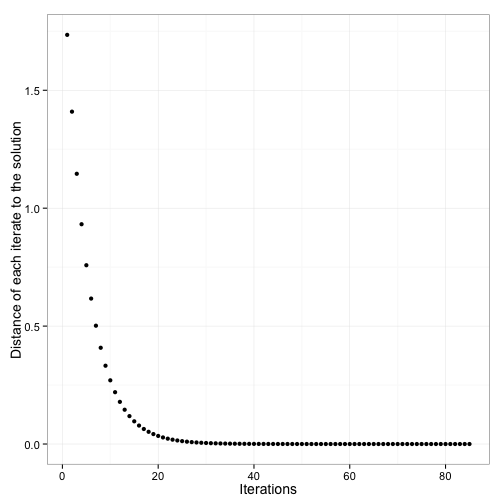#### Scaling the Variables.

In practice, gradient descent works best with objective functions that have very “round” level sets, and tends to perform slowly on functions with more elliptical level sets. For twice differentiable objective functions, this notion of eccentricity of level sets is captured by the condition number of the Hessian, or the ratio of the largest to the smallest eigenvalues of the Hessian. In the context of regression problems, ill-conditioned Hessians may arise when covariates contain values on drastically different scales. A natural remedy is to put all the columns of the design matrix $${\bf X}$$ on approximately the same scale. This process is also referred to as feature scaling, and can be achieved by standardization or even more simply, by dividing each element in $${\bf X}$$ by the maximal element in its column. The gdescent function performs feature scaling by default but one can also opt out of feature scaling by setting autoscaling=FALSE when calling the function. We provide an example of using gradient descent both with and without feature scaling later in this article.

#### A Summary of Simple Sanity Checks.

In view of the preceding considerations, we highlight a few possible indicators of necessary adjustment in implementing the gradient descent algorithm.

1. The function values are diverging.
– If the step-size is small enough and the objective function has a Lipschitz continuous gradient, the function values should always decrease with each iteration. If the function values are constantly increasing, the algorithm will not converge. This is an indicator that a smaller step-size should be selected. In the gdescent function, the plot_loss() function enables verification that the objective function values are decreasing over the course of the implementation.

2. The norm of the gradient is not approximately zero when the algorithm finishes running.
– We know that the gradient must vanish at a minimizer so if the norm of the gradient is not approximately zero, the algorithm has not minimized the function. In the gdescent function, the plot_gradient() function enables verification that the norm of the gradient is converging to zero.

3. The algorithm is taking a very long time to run when the number of covariates is not very large.
– This is not a guarantee that something is wrong with the implementation but it may be an indicator that the columns of the design matrix $${\bf X}$$ have not been scaled.

In this section, we discussed some of the key implementation considerations for the gradient descent algorithm. In the remaining section, we provide several more examples to illustrate the gradient descent algorithm in action.

## Examples.

#### Least Squares Regression with Gradient Descent.

The next two examples demonstrate use of the gradient descent algorithm to solve least squares regression problems.

In this first example, we use the moviebudgets dataset included in the gettingtothebottom package to estimate movie ratings based on their budgets. The dataset contains ratings and budgets for 5,183 movies. A quick look at the data shows us that the values in the budget and rating variables are on drastically different scales.

 data(moviebudgets)  head(moviebudgets)  

 ##                                title year length    budget rating votes  ## 1                            Titanic 1997    194 200000000    6.9 90195  ## 2                       Spider-Man 2 2004    127 200000000    7.9 40256  ## 3                               Troy 2004    162 185000000    7.1 33979  ## 4                         Waterworld 1995    176 175000000    5.4 19325  ## 5 Terminator 3: Rise of the Machines 2003    109 175000000    6.9 32111  ## 6                     Wild Wild West 1999    107 170000000    4.0 19078  ##     r1   r2   r3   r4   r5   r6   r7   r8   r9  r10  mpaa Action Animation  ## 1 14.5  4.5  4.5  4.5  4.5  4.5 14.5 14.5 14.5 24.5 PG-13      0         0  ## 2  4.5  4.5  4.5  4.5  4.5  4.5 14.5 24.5 24.5 24.5 PG-13      1         0  ## 3  4.5  4.5  4.5  4.5  4.5 14.5 14.5 14.5 14.5 14.5     R      1         0  ## 4  4.5  4.5  4.5 14.5 14.5 14.5 14.5 14.5  4.5  4.5 PG-13      1         0  ## 5  4.5  4.5  4.5  4.5  4.5 14.5 24.5 24.5  4.5 14.5     R      1         0  ## 6 14.5 14.5 14.5 14.5 14.5 14.5  4.5  4.5  4.5  4.5 PG-13      1         0  ##   Comedy Drama Documentary Romance Short  ## 1      0     1           0       1     0  ## 2      0     0           0       0     0  ## 3      0     1           0       1     0  ## 4      0     1           0       0     0  ## 5      0     0           0       0     0  ## 6      1     0           0       0     0  


We utilized the default feature scaling setting in the gdescent function to allow the algorithm to converge more quickly.

 # EXAMPLE 2 - Linear Regression & Feature Scaling   f <- function(X, y, b) {      (1/2) * norm(y - X %*% b, "F")^2      }  grad_f <- function(X, y, b) {      t(X) %*% (X %*% b - y)      }   X <- as.matrix(moviebudgets$budget) y <- as.vector(moviebudgets$rating)  movies1 <- gdescent(f, grad_f, X, y, 1e-04, 5000)  

 ## Minimum function value:  ##  6174  ##   ## Intercept:  ##  6.149  ##   ## Coefficient(s):  ##  -8.533e-10  


We can verify that these are the same results we get with the lm function.

lm(y ~ X) 
 ##   ## Call:  ## lm(formula = y ~ X)  ##   ## Coefficients:  ## (Intercept)            X    ##    6.15e+00    -8.53e-10  


Below is an example of the same problem without feature scaling. We observe that without feature scaling, the gradient descent algorithm requires a much smaller step-size and many more iterations. (In the interest of space, we do not include that output in this article.)

movies2 <- gdescent(f,grad_f,X,y,1e-19,10000,liveupdates=TRUE,autoscaling=FALSE) 

In the next example, we apply gradient descent to a multivariate linear regression problem using data from the baltimoreyouth dataset included in the gettingtothebottom package. Here, we want to predict the relationship between the percentage of students receiving free or reduced meals and the high school completion rate within each of the Community Statistical Areas (CSAs) in Baltimore. This model controls for the percentage of students suspended or expelled during the year, the percentage of students aged 16-19 who employed, and the percentage of students chronically absent in each CSA.

 # EXAMPLE 3 - Multivariate Linear Regression   f <- function(X, y, b) {      (1/2) * norm(y - X %*% b, "F")^2       }  grad_f <- function(X, y, b) {      t(X) %*% (X %*% b - y)       }   data(baltimoreyouth)  X <- matrix(c(baltimoreyouth$farms11, baltimoreyouth$susp11, baltimoreyouth$sclemp11, baltimoreyouth$abshs11), nrow = nrow(baltimoreyouth), byrow = FALSE)  y <- as.vector(baltimoreyouthcompl11) meals_graduations <- gdescent(f, grad_f, X, y, 0.01, 12000)    ## Minimum function value: ## 732.2 ## ## Intercept: ## 93.93 ## ## Coefficient(s): ## -0.03576 -0.11384 -0.05470 -0.13388   Again, we can compare the results from gradient descent to those from the lm function. lm(y ~ X)   ## ## Call: ## lm(formula = y ~ X) ## ## Coefficients: ## (Intercept) X1 X2 X3 X4 ## 93.9258 -0.0358 -0.1138 -0.0547 -0.1339   #### Fitting Logistic Regression with Gradient Descent As our last example, we consider standard logistic regression. We have a response $${\bf y}$$ that is binary, namely $${\bf y} = \begin{pmatrix} y_{1} & y_{2} & \dots & y_{n} \end{pmatrix}^{t}$$ with $$y_{i} \in \{0,1\}$$ for $$i = 1, \ldots, n$$. Let $${\bf X} \in \mathbb{R}^{n \times p}$$ denote a set of covariates, and as before we want to estimate a regression vector $${\bf b} \in \mathbb{R}^{p}$$. In the logistic model, we assume the probability of observing $$y_{i} = 1$$ given $${\bf Xb}$$ is given by \begin{align*} P(y_{i}=1 | {\bf Xb}) = \frac{\exp({\bf x_{i}}^{t}{\bf b})}{1+ \exp({\bf x_{i}}^{t}{\bf b})}. \end{align*} If $${\bf x_{i}}^{t}{\bf b}$$ is very positive, the probability will be close to $$1$$, and if it is very negative, then the probability will be close to $$0$$. We estimate the regression vector $${\bf b}$$ by maximizing the log-likelihood or equivalently minimizing the negative log-likelihood $$\ell({\bf b})$$, which is given by \begin{align*} \ell({\bf b}) = \sum_{i=1}^{n}\left( -y_{i}{\bf x_{i}}^{t}{\bf b} + \log[1+\exp({\bf x_{i}}^{t}{\bf b})]\right). \end{align*} Then the gradient of $$\ell({\bf b})$$ is \begin{align*} \nabla \ell({\bf b}) = -{\bf X}^{t}({\bf y}-{\bf p}), \end{align*} where $${\bf p}$$ is the vector of fitted responses, namely $$p_{i} = \frac{1}{1+e^{-{\bf x_{i}}^{t}{\bf b}}}$$. We observe that the estimating equations $$\nabla \ell({\bf b}) = {\bf 0}$$ are nonlinear and consequently, we must resort to an iterative algorithm to obtain the maximum likelihood estimate. Note that the Hessian of $$\ell({\bf b})$$ is given by \begin{align*} \nabla^2 \ell({\bf b}) = {\bf X}^t{\bf W}{\bf X}, \end{align*} where $${\bf W}$$ is a diagonal matrix whose $$i^{\text{th}}$$ entry is given by $$w_i = p_i(1-p_i)$$. It is not hard to show that the largest eigenvalue of $$\nabla^2 \ell({\bf b})$$ is bounded from above by $$1/4$$ times the largest eigenvalue of $${\bf X}^t{\bf X}$$. In the following example with simulated data, we use this bound to choose our step-size.  # EXAMPLE 4 - Logistic Regression set.seed(12345) n <- 100 p <- 10 X <- matrix(rnorm(n * p), n, p) b <- matrix(rnorm(p), p, 1) e <- 0.5 * matrix(rnorm(n), n, 1) z <- X %*% b + e y <- as.vector((plogis(z) <= runif(n)) + 0) l <- function(X, y, b) { -t(y) %*% (X %*% b) + sum(log(1 + exp(X %*% b))) } grad_l <- function(X, y, b) { -t(X) %*% (y - plogis(X %*% b)) } alpha = 4/(svd(cbind(1, X))d^2)  # Use gradient descent algorithm to solve logistic regression problem  logistic_ex <- gdescent(l, grad_l, X, y, alpha = alpha, iter = 15000)  

 ## Minimum function value:  ##  22.34  ##   ## Intercept:  ##  -0.6918  ##   ## Coefficient(s):  ##  -3.06784 -0.67156 1.97324 1.36163 0.42671 3.77991 0.04290 2.29480 -0.01141 -0.60526  


We can plot the values of the objective function to verify convergence of our algorithm.

plot_loss(logistic_ex)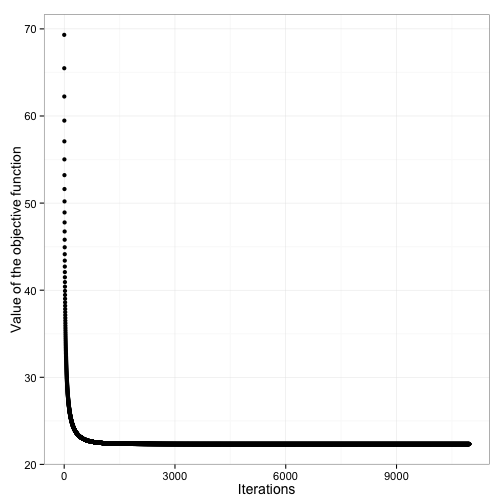We can also compare our results with those obtained from the glm function and observe that the gradient descent algorithm provides the same solution.

 # Use glm function to solve logistic regression problem  glm(y ~ X, family = binomial)  

 ##   ## Call:  glm(formula = y ~ X, family = binomial)  ##   ## Coefficients:  ## (Intercept)           X1           X2           X3           X4    ##     -0.6918      -3.0678      -0.6716       1.9732       1.3616    ##          X5           X6           X7           X8           X9    ##      0.4267       3.7799       0.0429       2.2948      -0.0114    ##         X10    ##     -0.6053    ##   ## Degrees of Freedom: 99 Total (i.e. Null);  89 Residual  ## Null Deviance:       139   ## Residual Deviance: 44.7  AIC: 66.7  


## Conclusion

In this article, we saw that the gradient descent algorithm is an extremely simple algorithm. Much more can be said about its theoretical properties, but our aim in this article was to highlight the basic mechanics, intuition, and biggest practical issues in implementing gradient descent so that you might be able to use it in your work. Many fine texts delve further in details we did not have space to explore here, and in particular, we point interested readers to Numerical Optimization by Nocedal and Wright and Numerical Analysis for Statisticians by Lange for a more thorough treatment.

## Books & Journals

### Books#### Common Errors in Statistics (and How to Avoid Them), 4th Edition#### The Analysis of Covariance and Alternatives: Statistical Methods for Experiments, Quasi-Experiments, and Single-Case Studies, 2nd EditionView all

### Journals#### Mathematical Finance#### ComplexityView all